# Cantilever Beam with Eccentric Point MassesThe purpose of this test is to check the coupling between torsional and flexural behavior. You will use a 1D beam.Reference:

NAFEMS-Glasgow, Benchmark newsletter, p.15, April 1989.

##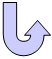Specifications

### Geometry Specifications

 Length: L = 10 m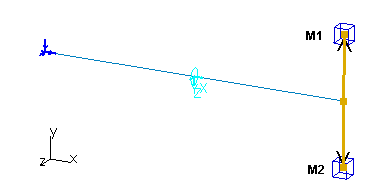Section (circular): D = 0.5 m Mass 1 (center): x = 10 m y = 2 m M1 = 10000 kg Mass 2 (center):   x = 10 m y = 2 m M2 = 1000 kg

### Analysis Specifications

 Young Modulus (material): E = 200 GPa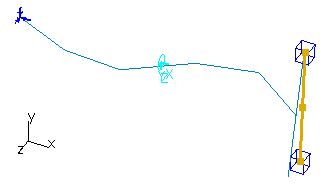View of the mode 7 Poisson's Ratio (material): ν = 0.3 Mesh Specifications: 5 beam elements along the cantilever To define the rigid bar between the two masses,   use a General Analysis Connection and a User-defined Connection Property (Rigid as Middle option). Restraints (User-defined): At end 1, Tx = Ty = Tz = Rx = Ry = Rz = 0

##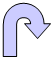Results

The results are based on the 6 lowest normal modes of the structure.
The results and the percentage of errors are listed in the table below:

 Mode Exact [Hz] Beam [Hz] Error [%] 1 1.723 1.722 0.058 2 1.727 1.725 0.116 3 7.413 7.410 0.040 4 9.972 9.946 0.261 5 18.160 18.046 0.628 6 26.972 26.697 1.020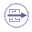To Perform the Test:

The cantilever_beam_excentric_point_masses.CATAnalysis document presents a complete analysis of this case, computed with a mesh formed of beam elements. Proceed as follow:

1. Open the CATAnalysis document.

2. Compute the case and generate an image called Deformed mesh.